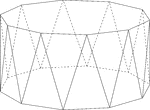Decagonal Antiprism

Illustration of a decagonal antiprism. An antiprism is formed by having two parallel congruent bases…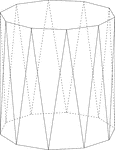Decagonal Antiprism

Illustration of a decagonal antiprism. An antiprism is formed by having two parallel congruent bases…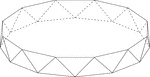Dodecagonal Antiprism

Illustration of a dodecagonal antiprism. An antiprism is formed by having two parallel congruent bases…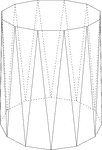Dodecagonal Antiprism

Illustration of a dodecagonal antiprism. An antiprism is formed by having two parallel congruent bases…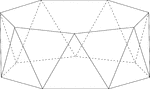Heptagonal/Septagonal Antiprism

Illustration of a heptagonal, or sometimes known as a septagonal antiprism. An antiprism is formed by…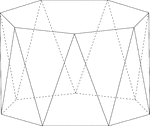Heptagonal/Septagonal Antiprism

Illustration of a heptagonal, or sometimes known as a septagonal antiprism. An antiprism is formed by…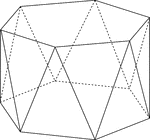Hexagonal Antiprism

Illustration of a hexagonal antiprism. An antiprism is formed by having two parallel congruent bases…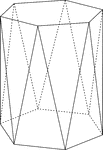Hexagonal Antiprism

Illustration of a hexagonal antiprism. An antiprism is formed by having two parallel congruent bases…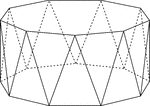Nonagonal Antiprism

Illustration of a nonagonal antiprism. An antiprism is formed by having two parallel congruent bases…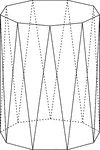Nonagonal Antiprism

Illustration of a nonagonal antiprism. An antiprism is formed by having two parallel congruent bases…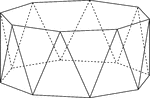Octagonal Antiprism

Illustration of an octagonal antiprism. An antiprism is formed by having two parallel congruent bases…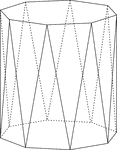Octagonal Antiprism

Illustration of an octagonal antiprism. An antiprism is formed by having two parallel congruent bases…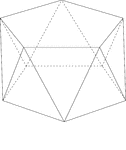Pentagonal Antiprism

Illustration of a pentagonal antiprism. An antiprism is formed by having two parallel congruent bases…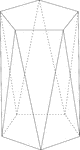Pentagonal Antiprism

Illustration of a pentagonal antiprism. An antiprism is formed by having two parallel congruent bases…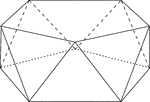Pentagonal Antiprism

Illustration of a pentagonal antiprism. An antiprism is formed by having two parallel congruent bases…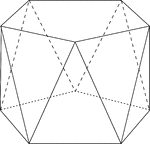Pentagonal Antiprism

Illustration of a pentagonal antiprism. An antiprism is formed by having two parallel congruent bases…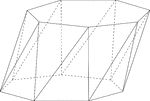Skewed Heptagonal/Septagonal Antiprism

Illustration of a skewed (non-right) heptagonal, or sometimes known as a septagonal antiprism. An antiprism…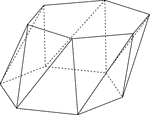Skewed Hexagonal Antiprism

Illustration of a skewed (non-right) hexagonal antiprism. An antiprism is formed by having two parallel…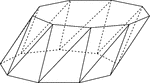Skewed Nonagonal Antiprism

Illustration of a skewed (non-right) nonagonal antiprism. An antiprism is formed by having two parallel…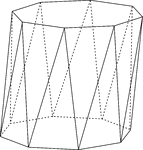Skewed Octagonal Antiprism

Illustration of a skewed (non-right) octagonal antiprism. An antiprism is formed by having two parallel…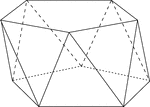Skewed Pentagonal Antiprism

Illustration of a skewed (non-right) pentagonal antiprism. An antiprism is formed by having two parallel…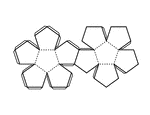Pattern for Dodecahedron

Pattern that can be cut out and folded to construct a regular dodecahedron. Fold on the dotted lines,…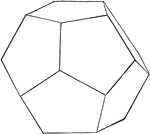Regular Dodecahedron

Illustration of a regular dodecahedron. A regular dodecahedron is a polyhedron with twelve equal pentagonal…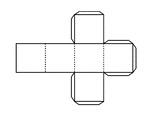Pattern for Hexahedron

Pattern that can be cut out and folded to construct a regular hexahedron. Fold on the dotted lines,…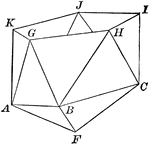Part of an Icosahedron

Illustration of the bottom part of an icosahedron. The base consists of a regular pyramid, upon which…Partial Construction of an Icosahedron

Illustration of the steps to a construction of an icosahedron. The base consists of a regular pyramid,…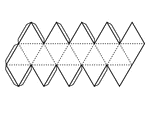Pattern for Icosahedron

Pattern that can be cut out and folded to construct a regular icosahedron. Fold on the dotted lines,…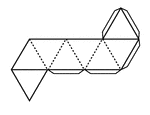Pattern for Octahedron

Pattern that can be cut out and folded to construct a regular octahedron. Fold on the dotted lines,…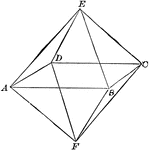Regular Octahedron

Illustration of a regular octahedron. A polyhedron with eight equal faces.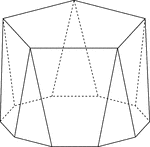Polyhedron With Pentagon Bases

Illustration of a pentagonal polyhedron that is formed by having two parallel congruent pentagonal bases…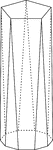Polyhedron With Pentagon Bases

Illustration of a pentagonal polyhedron that is formed by having two parallel congruent pentagonal bases…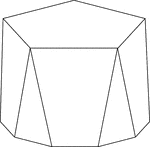Polyhedron With Pentagon Bases

Illustration of a pentagonal polyhedron that is formed by having two parallel congruent pentagonal bases…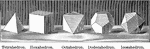Regular Polyhedrons

Illustration of regular polyhedrons: tetrahedron, hexahedron, octahedron, dodecahedron, icosahedron.Similar Polyhedrons

Two similar polyhedrons may be decomposed into the same number of tetrahedrons similar, each to each,…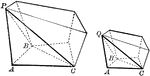Two Similar Polyhedrons

Diagram used to prove the theorem: "Two similar polyhedrons may be decomposed into the same number of…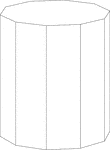Decagonal Prism

Illustration of a right decagonal prism with regular decagons for bases and rectangular faces.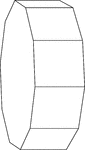Decagonal Prism Resting On Its Side

Illustration of a right decagonal prism with regular decagons for bases and rectangular faces. The prism…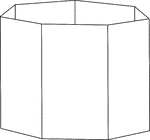Heptagonal/Septagonal Prism

Illustration of a hollow right heptagonal/septagonal prism with regular heptagons/septagons for bases…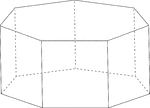Heptagonal/Septagonal Prism

Illustration of a regular right heptagonal/septagonal prism with regular heptagons/septagons for bases…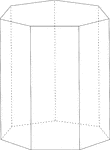Heptagonal/Septagonal Prism

Illustration of a right heptagonal/septagonal prism with regular heptagons/septagons for bases and rectangular…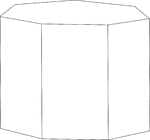Heptagonal/Septagonal Prism

Illustration of a right heptagonal/septagonal prism with regular heptagons/septagons for bases and rectangular…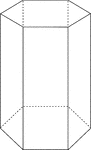Hexagonal Prism

Illustration of a right hexagonal prism with hidden edges shown.Hexagonal Prism

Illustration of a right hexagonal prism with a height less than the length of the edge of the hexagon.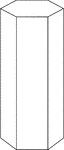Hexagonal Prism

Illustration of a right hexagonal prism with a height greater than the length of the edge of the hexagon.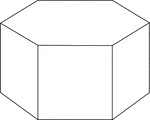Hexagonal Prism

Illustration of a right hexagonal prism with hexagons for bases and square faces.Hexagonal Prism

Illustration of a right hexagonal prism with hexagons for bases and square faces. The hidden edges are…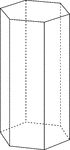Hexagonal Prism

Illustration of a right hexagonal prism with hexagons for bases and rectangular faces. The height of…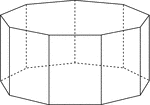Nonagonal Prism

Illustration of a right nonagonal prism with regular nonagons for bases and rectangular faces. The hidden…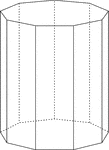Nonagonal Prism

Illustration of a right nonagonal prism with regular nonagons for bases and rectangular faces. The height…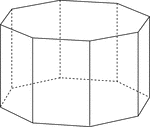Octagonal Prism

Illustration of a right octagonal prism with regular octagons for bases and rectangular faces. The hidden…Octagonal Prism

Illustration of a right octagonal prism with regular octagons for bases and rectangular faces. The height…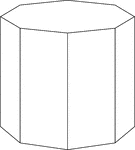Octagonal Prism

Illustration of a right octagonal prism with octagons for bases and rectangular faces. The height of…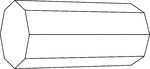Octagonal Prism Resting On Side

Illustration of a right octagonal prism, with octagons for bases and rectangular faces, that is resting…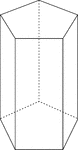Pentagonal Prism

Illustration of a right pentagonal prism with regular pentagons for bases and rectangular faces. The…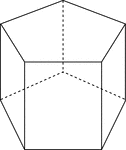Pentagonal Prism

Illustration of a right pentagonal prism with regular pentagons for bases and rectangular faces. The…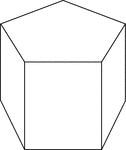Pentagonal Prism

Illustration of a right pentagonal prism with regular pentagons for bases and rectangular faces. The…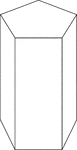Pentagonal Prism

Illustration of a right pentagonal prism with regular pentagons for bases and rectangular faces. The…2 Octagonal Prisms

Illustration of 2 right octagonal prisms with congruent bases, but different heights. The height of…